###Author Topic: Sep 16, 2018 - Rendering Planetoids  (Read 1157 times)

#### ArenMook

• Hero Member
•• Thank You
• -Given: 337
• Posts: 22,154##### Sep 16, 2018 - Rendering Planetoids
« on: September 16, 2018, 05:13:37 PM »
As part of R&D for Project 5: Sightseer, I was looking into various ways of replacing Unity's terrain with something more flexible. Among my options was revisiting the planetary rendering system I wrote two years ago. Of course adapting a spherical planetary terrain to replace a flat one of a game that has been in development for 2.5 years is probably not the best idea in itself... and I'm still on the fence about using it... but I did get something interesting out of it: a way to generate planetoids.

The original idea was inspired by a Star Citizen video from a few years back where one of the developers was editing a planetary terrain by basically dragging splat textures onto it -- mountains and such, and the system would update immediately. Of course nothing like that exists in Unity, or on its Asset Store, so the only way to go was to try developing this kind of system myself.

So I started with a simple thought... what's the best way to seamlessly texture a sphere? Well, to use a cube map of course! A cube map is just a texture projected to effectively envelop the entire sphere without seams, so if one was to render a cube map from the center of the planet, it would be possible to add details to it in form of textured quads.

I started with a simple GPU-generated simplex noise shader that uniformly textured my sphere.Doesn't look like much, but that's just the source object that's supposed to act as a spherical height map. Now taking that and rendering it into a cube map gives a cube map heightmap that can then be used to texture the actual planet. Of course using the rendered cube map as-is wouldn't look very good. It would simply be the same sphere as above, but with lighting applied to it. More work is needed to get it to look more interesting.

First -- this is a height map, so height displacement should happen. This is simple enough to do just by displacing the vertex along the normal based on the sampled height value in the vertex shader.

1. v.vertex.xyz += v.normal * texCUBElod(_Cube, float4(v.normal, 0.0)).r * _Displacement;

This adds some height variance to the renderer, and combined with the basic assignment of the sampled texture to Albedo this makes the planetoid a little bit more interesting:Of course the lighting is not correct at this point. Although the vertex values get offset, normals do not. Plus, the vertex resolution is a lot lower than the source height map texture. So what ideally should happen, is the normal map should get calculated at run-time by sampling the height map values around each pixel. This process is different from the normal bump mapping technique because our texture is cubic rather than 2D. In a way, it's actually more simple. The challenge, as I learned, lies in calculating the normal itself.

With 2D textures, calculating normals from a heightmap is trivial: sample the height difference on +X to -X, then another height difference on +Y to -Y, use that as X and Y normal map, with Z being resolved based on the other two. With triangle-based meshes this is also simple: loop through triangles, calculate each triangle's normal, add to each of the 3 vertices, then normalize the result at the end. But with cube map textures? There is technically no left/right or up/down. Unprojecting each of the 6 faces would result in 2D textures, but then they wouldn't blend correctly with their neighbors. I spent a lot of time trying to generate the "perfect" normal map from a cube map heightmap texture, and in the end never got it to be quite perfect. I think the best solution would be to handle sides (+X, -X, +Z, -Z) and top/bottom (+Y, -Y) separately, then blend the result, but in my case I just did it without blending, simply using each normal along with the side's tangent (a simple right-pointing vector that I pass to each side's shader) to calculate the binormal. I then use the tangent and binormal to rotate the normal, thus creating the 4 sampling points used to calculate the modified normal.
1. inline float4 AngleAxis (float radians, float3 axis)
2. {
3.         radians *= 0.5;
4.         axis = axis * sin(radians);
5.         return float4(axis.x, axis.y, axis.z, cos(radians));
6. }
7.
8. inline float3 Rotate (float4 rot, float3 v)
9. {
10.         float3 a = rot.xyz * 2.0;
11.         float3 r0 = rot.xyz * a;
12.         float3 r1 = rot.xxy * a.yzz;
13.         float3 r2 = a.xyz * rot.w;
14.
15.         return float3(
16.                 dot(v, float3(1.0 - (r0.y + r0.z), r1.x - r2.z, r1.y + r2.y)),
17.                 dot(v, float3(r1.x + r2.z, 1.0 - (r0.x + r0.z), r1.z - r2.x)),
18.                 dot(v, float3(r1.y - r2.y, r1.z + r2.x, 1.0 - (r0.x + r0.y))));
19. }
20.
21. inline float SampleHeight (float3 normal) { return texCUBE(_Cube, normal).r; }
22.
23. float3 CalculateNormal (float3 n, float4 t, float textureSize)
24. {
25.         float pixel = 3.14159265 / textureSize;
26.         float3 binormal = cross(n, t.xyz) * (t.w * unity_WorldTransformParams.w);
27.         float3 x0 = Rotate(AngleAxis(-pixel, binormal), n);
28.         float3 x1 = Rotate(AngleAxis(pixel, binormal), n);
29.         float3 z0 = Rotate(AngleAxis(-pixel, t.xyz), n);
30.         float3 z1 = Rotate(AngleAxis(pixel, t.xyz), n);
31.
32.         float4 samp;
33.         samp.x = SampleHeight(x0);
34.         samp.y = SampleHeight(x1);
35.         samp.z = SampleHeight(z0);
36.         samp.w = SampleHeight(z1);
37.         samp = samp * _Displacement + 1.0;
38.
39.         x0 *= samp.x;
40.         x1 *= samp.y;
41.         z0 *= samp.z;
42.         z1 *= samp.w;
43.
44.         float3 right = (x1 - x0);
45.         float3 forward = (z1 - z0);
46.         float3 normal = cross(right, forward);
47.         normal = normalize(normal);
48.
49.         if (dot(normal, n) <= 0.0) normal = -normal;
50.         return normal;
51. }
The world normal is calculated in the fragment shader and is then used in the custom lighting function instead of the .Normal.
1. float3 worldNormal = normalize(IN.worldNormal);
2. float3 objectNormal = normalize(mul((float3x3)unity_WorldToObject, worldNormal));
3. float height = SampleHeight(objectNormal);
4. objectNormal = CalculateNormal(objectNormal, _Tangent, 2048.0);
5. o.WorldNormal = normalize(mul((float3x3)unity_ObjectToWorld, objectNormal));
6. o.Albedo = height.xxx;
7. o.Alpha = 1.0;
The result looks like this:Now in itself, this is already quite usable as a planetoid / asteroid for space games, but it would be great to add some other biomes, craters and hills to it. The biomes can be done by changing the noise shader, or by adding a second noise on top of the first one, that will alpha blend with the original. Remember: in our case the height map texture is a rendered texture, so we can easily add additional alpha-blended elements to it, including an entire sphere.

This is how a secondary "biome" sphere looks like that adds some lowlands:Blended together it looks like this on the source sphere:When rendered into a cube map and displayed on the planetoid, it looks like this: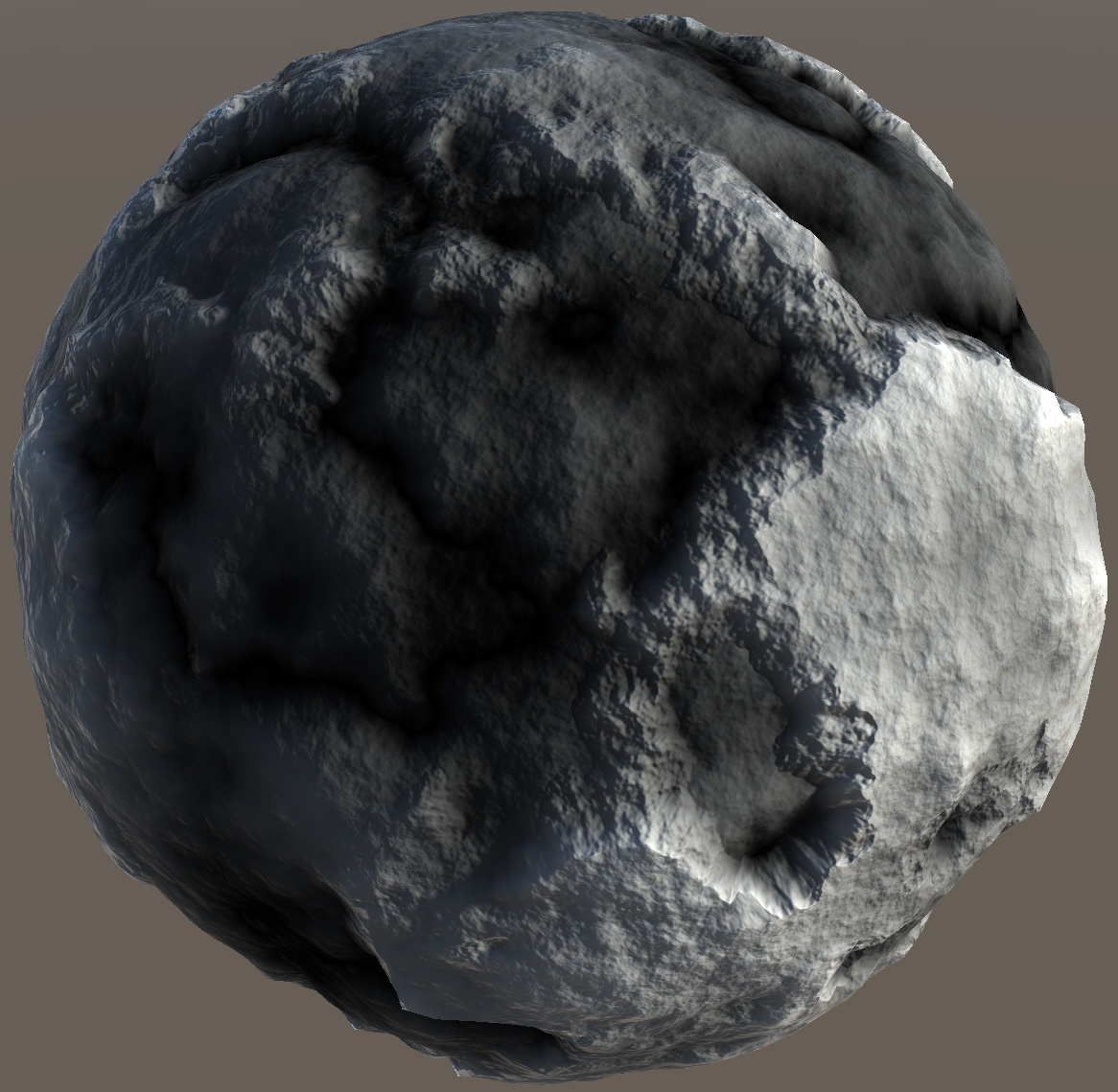Adding craters and other features to the surface at this point is as simple as finding good height map textures, assigning them to a quad, and placing them on the surface of the sphere:The final result looks excellent from far away: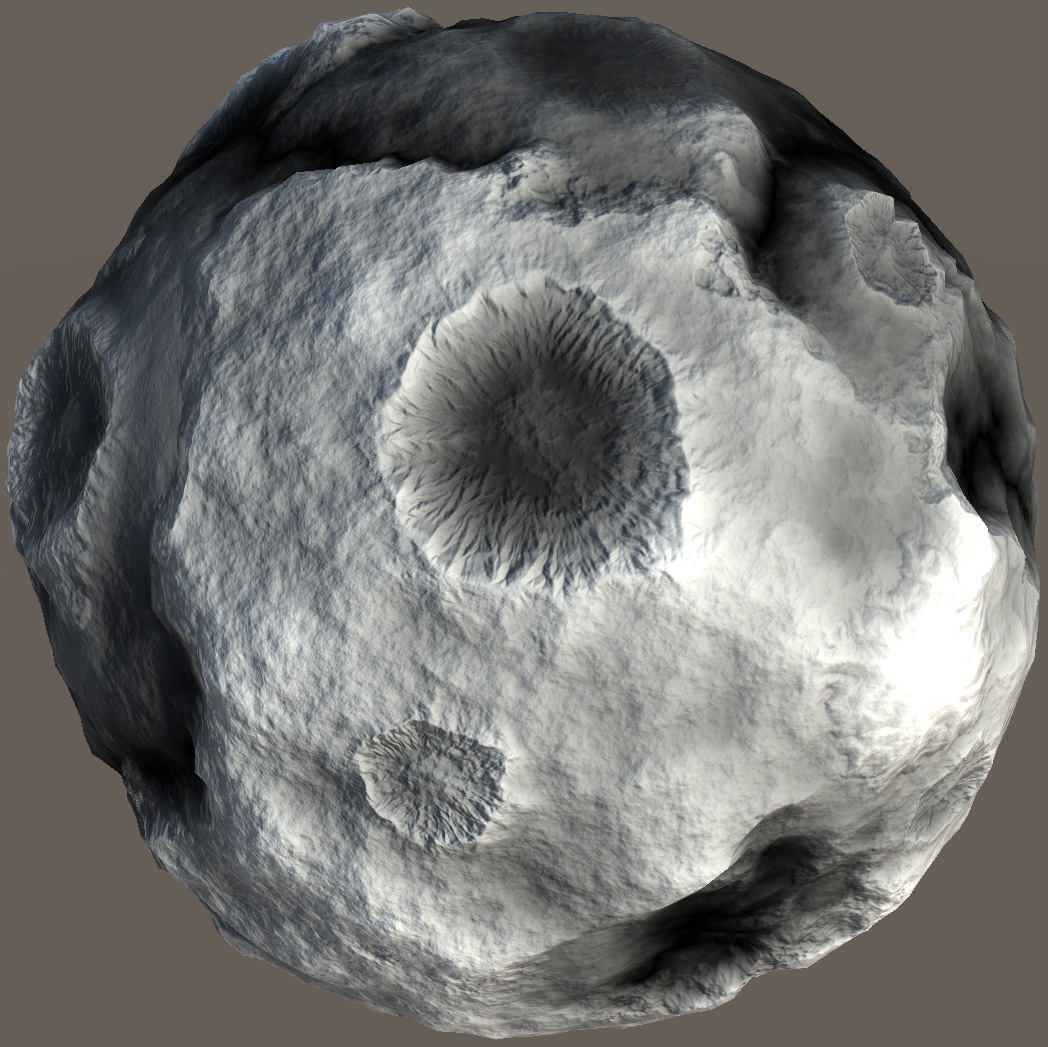Unfortunately zooming in, especially in areas with flatter terrain, obvious ridges form: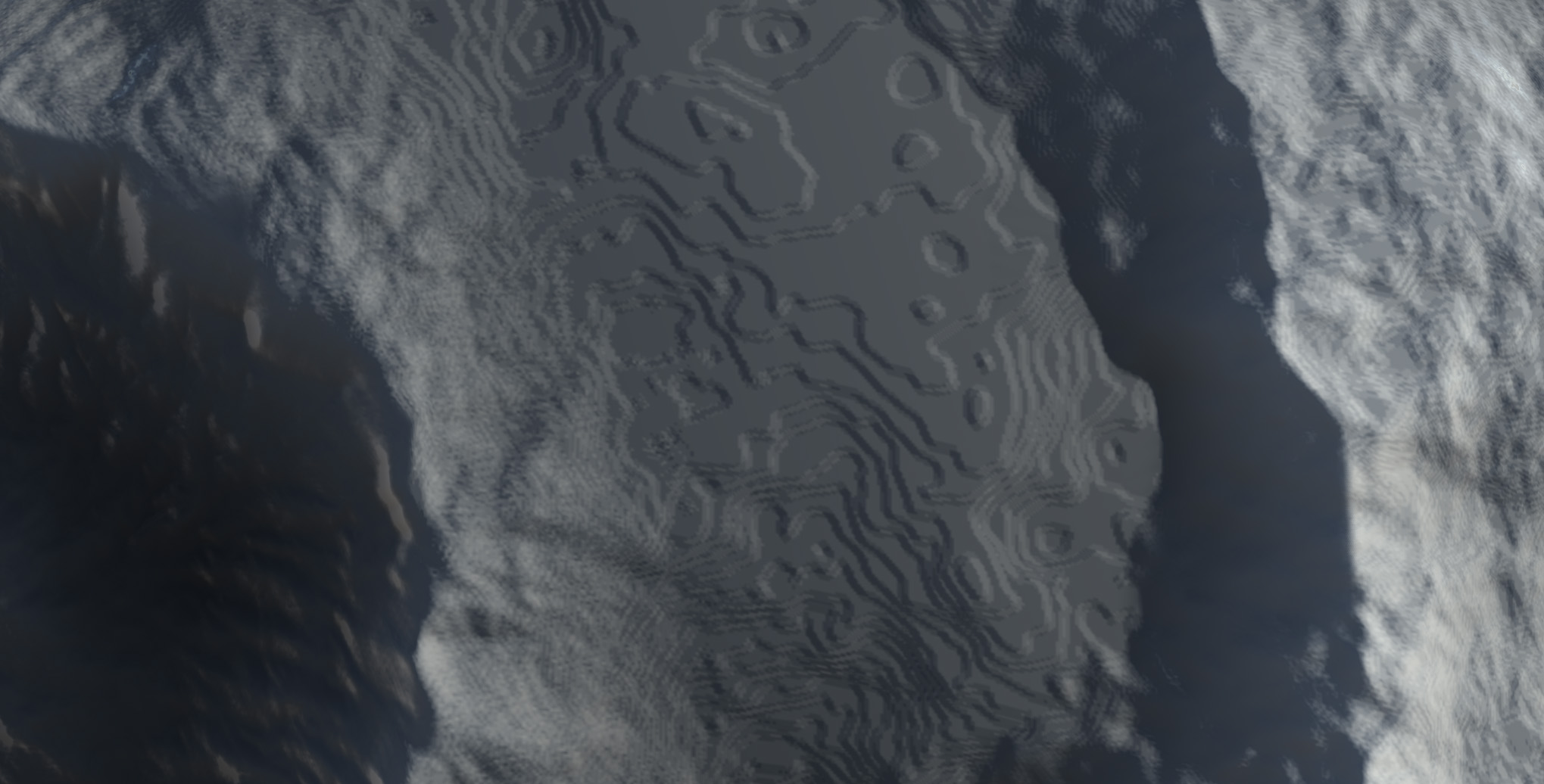This happens because 8-bit colors are simply inadequate when it comes to handling the height variance found in terrains. So what can be done? The first obvious thing I tried was to pack the height value into 2 channels using Unity's EncodeFloatRG function in the original biome shaders:
1. // Instead of this:
2. return half4((noise * 0.5 + 0.5).xxx, 1.0);
3. // I did this:
4. return half4(EncodeFloatRG(noise * 0.5 + 0.5), 0.0, 1.0);
The SampleHeight function was then changed to:
1. inline float SampleHeight (float3 normal) { return DecodeFloatRG(texCUBE(_Cube, normal).rg); }
This certainly helped the biomes look smooth, but the stamp textures (craters, mountains, etc) are still regular textures, so there is obviously no benefit to taking this approach with them. Worse still, the alpha blending is still 8 bit! You can see that the transition walls (the slope on the right side of the picture below) are still pixelated, because alpha blending doesn't have the advantage of taking the 16-bit approach of EncodeFloatRG.Worse still, the source sphere became unrecognizable: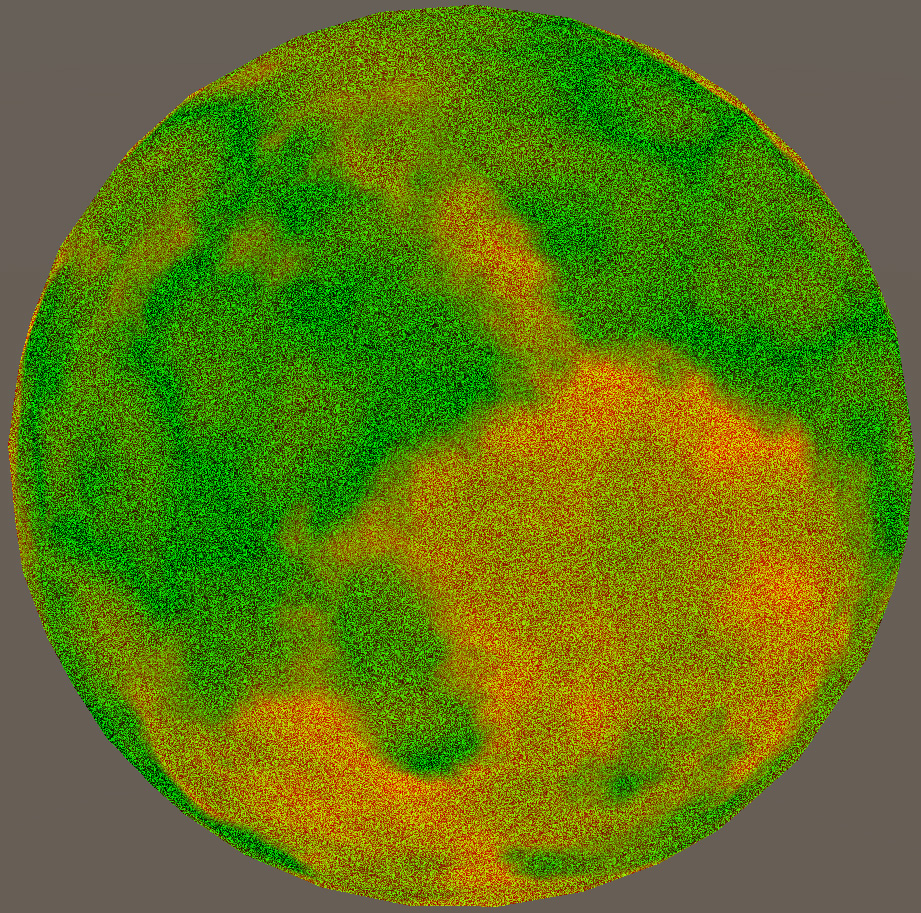So what can be done to fix this? Well, since the stamp source heightmap textures are always using 8 bits per color, there isn't a whole lot that can be done here, short of creating your own custom heightmaps and saving them in 16-bit per color textures, which sadly means ruling out all the height maps readily available online like the ones I used. The transitions between biomes can be eliminated by using only a single shader that would contain all the biomes and blend them together before outputting the final color in RG format. So, technically if one was to avoid stamp textures, it's possible to have no visible jaggies.

At this point, some of you reading may wonder, "why don't you just change the render texture format to float, you noob?" -- I tried... Never mind the RGBA format 2048 size cube map is already 128 MB in size, there is no option to make it be anything else. It's not possible to make it bigger, and it's not possible to change it to be anything other than RGBA: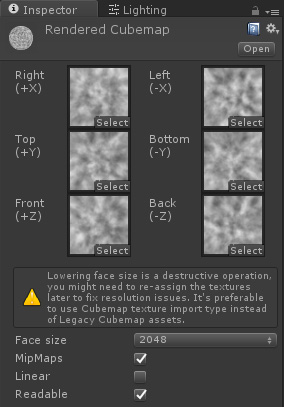I tried doing this via code -- RFloat format flat out failed. Unity did seem to accept the RGBAFloat format, but then the final texture was always black, even though there was no errors or warnings in the console log. Plus, the texture size of 512 MB was kind of... excessive to begin with. And so what's left to try at this point? I suppose I could change the process to render 6 2D textures instead of the single cube map, which would allow me to use the RFloat format, but this will also mean visible seams between the quadrants, since the process of generating normals won't be able to sample adjacent pixels.

I could also try using the RGBAFloat format, rendering height into R, and normal XY into G & B channels. This would, in theory, be the best approach -- as the final planetoid won't need to keep calculating normal maps on the fly. Of course the memory usage at this point will be 512 MB per planetoid with texture resolution of 2048... So again back to being rather excessive.

If you have any thoughts on the matter, and especially if you've done this kind of stuff before, let me know! I can be found on the Tasharen's Discord.
« Last Edit: September 16, 2018, 05:21:27 PM by ArenMook »